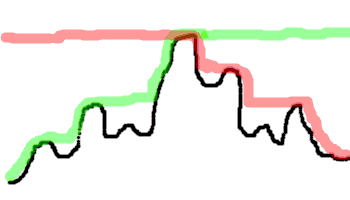http://puzzles.bostonpython.com/puddle.html

Solutions by Steve Witham.

• The first is based on a simple declarative definition of water depth.
• The second is an optimization of the first.
• The third is a stack-based solution for speed and memory use comparison.
In :
# Set up the list of problem examples and the list of methods.
# Methods and more problems get added to the lists later.

from collections import OrderedDict

EXAMPLES = []

EXAMPLES.append( ("Empty", [], 0) )

"""
_   _   _
|2|w|2|w|2|_
|__1___1___1|
0 1 2 3 4 5
"""
EXAMPLES.append( ("Two puddles same height", [2, 1, 2, 1, 2, 1], 2) )
"""
_ _
|7 7|_
_        |    6|
|5|w w w w|     |
| |w w w|4      |
_| |w w|3        |
|2  |w|2          |
|____1____________|
0 1 2 3 4 5 6 7 8
"""
EXAMPLES.append( ("Original", [2, 5, 1, 2, 3, 4, 7, 7, 6], 10) )

METHODS = OrderedDict()
def METHOD(method):
" A decorator to register OR REREGISTER a method function in METHODS. "
METHODS[method.__name__] = method
return method


### Cumulative Maxes to the Left and Right¶

The height of the water surface above a given block is the lower of

• the highest wall to the left (including this block) and
• the highest wall to the right (including this block).In :
from itertools import izip

def cumulative_maxes(ys):
m = None
for y in ys:
m = max(m, y)
yield m

@METHOD
def puddle_vol_cumulative_maxes(ys):
"""
This relies on ys being a list, and uses an equal amount of extra memory.
"""
maxes_to_L = cumulative_maxes(ys)
maxes_to_R = reversed(list(cumulative_maxes(reversed(ys))))
return sum(min(mL, mR) - y for mL, y, mR in izip(maxes_to_L, ys, maxes_to_R))


### Find the Peak, then Climb the Slopes¶

In :
@METHOD
def puddle_vol_peak_then_slopes(ys):
"""
Once you know the max of the whole ys list, and its position in the list
(if the max appears in more than one place, it doesn't matter which you pick),
then water heights on its left only depend on wall heights to their left,
and water heights on its right only depend on wall heights to their right.

This depends on ys being a list, but creates no other lists.
"""
if not ys: return 0  #  Can't take max of an empty list.

peak_i = ys.index(max(ys))
vol = 0
for slope in xrange(peak_i), xrange(len(ys) - 1, peak_i, -1):
highest = None
for i in slope:
y = ys[i]
highest = max(highest, y)
vol += highest - y
return vol


### Strictly Left-to-Right with a Stack¶

In :
@METHOD
def puddle_vol_L_to_R_stack(ys):
"""
This is a stack-based solution for speed and space comparison.
Each stack entry locates the upper-right square of a rectangle.
x's of Points on the stack are strictly increasing; y's strictly decreasing.

This does not depend on ys being a list.
The size of the stack can be twice the size of ys.
"""
stack_x, stack_y = [], []
vol = 0
for cx, cy in enumerate(ys):
while len(stack_y) >= 2 and stack_y[-1] < cy:
bx, by = stack_x.pop(), stack_y.pop()
ax, ay = stack_x[-1], stack_y[-1]
vol += (min(ay, cy) - by) * (cx - ax - 1)
if ay < cy:
stack_x[-1] = bx
if stack_y and stack_y[-1] <= cy:
stack_x.pop(), stack_y.pop()
stack_x.append(cx)
stack_y.append(cy)
return vol


### Testing and Timing¶

In :
from time import clock
import random

def make_problems(n_problems, n_heights):
problems = []
for i in range(1, n_problems + 1):
name = "random problem %d" % i
heights = [random.randrange(1, n_heights + 1) for j in range(n_heights)]
correct_vol = None
problems.append( (name, heights, correct_vol) )
return problems

def make_example_pairs(examples):
example_pairs = []
for example in examples:
name, heights, correct_vol = example
rev_heights = list(reversed(heights))
rev_example = ("reversed " + name, rev_heights, correct_vol)
example_pairs.append( (example, rev_example) )
return example_pairs

def test(methods, examples, n_repeats=100):
example_pairs = make_example_pairs(examples)
results = []
for method in METHODS.values():
start = clock()
for example_pair in example_pairs:
for example in example_pair:
name, heights, correct_vol = example
for i in range(n_repeats):
duration = (clock() - start) / n_repeats
return results

def show_results(results):
for method, answer_pairs, duration in results:
print method.__name__
n_mistakes = 0
n_mismatches = 0
problem_name, heights, correct_vol = problem
if correct_vol != None and answer != correct_vol:
n_mistakes += 1
n_mismatches += 1
if n_mistakes:
print "   ", n_mistakes, "mistakes"
if n_mismatches:
print "   ", n_mismatches, "disagreements with", results.__name__
print "   ", duration, "sec."

random.seed(201406271651)
problem_set = EXAMPLES + make_problems(n_problems=100, n_heights=100)
results = test(METHODS, problem_set, n_repeats=10)
show_results(results)

puddle_vol_max_L_max_R
0.0644718 sec.
puddle_vol_peak_then_slopes
0.0244862 sec.
puddle_vol_L_to_R_stack
0.1103731 sec.

In [ ]: